Electron. J. Diff. Eqns., Vol. 2005(2005), No. 106, pp. 1-18.

### Positive solutions of three-point boundary-value problems for p-Laplacian singular differential equations George N. Galanis, Alex P. Palamides

Abstract:
In this paper we prove the existence of positive solutions for the three-point singular boundary-value problem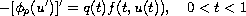subject to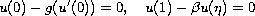or to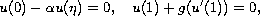where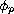is the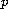-Laplacian operator,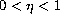;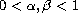are fixed points andis a monotone continuous function defined on the real line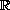with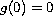and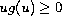. Our approach is a combination of Nonlinear Alternative of Leray-Schauder with the properties of the associated vector field at the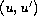plane. More precisely, we show that the solutions of the above boundary-value problem remains away from the origin for the case where the nonlinearity is sublinear and so we avoid its singularity at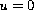.

Submitted May 13, 2005. Published October 7, 2005.
Math Subject Classifications: 34B15, 34B18.
Key Words: Three-point singular boundary-value problem; p-Laplacian; positive and negative solutions; vector field; Nonlinear alternative of Leray-Schauder.

Show me the PDF file (292K), TEX file, and other files for this article.George N. Galanis Naval Academy of Greece Piraeus, 185 39, Greece email: ggalanis@math.uoa.gr Alex P. Palamides Department of Communication Sciences University of Peloponnese 22100 Tripolis, Greece email: palamid@uop.gr